## Parameters Diverging to $\pm \infty$ in Logistic Regression¶

#### Suraj Rampure (suraj.rampure@berkeley.edu)¶

Updated May 15, 2019

Motivation: There have been several questions, both in OH and on Piazza, regarding the divergence of parameters in logistic regression when our data is linearly separable. This notebook is meant to provide some sort of intuition, but doesn't walk through any math rigorously.

For this notebook, suppose our model is $$P(Y = 1 \: \big| \:X = x) = \sigma(\beta_0 + \beta_1x) = \frac{1}{1 + e^{-(\beta_0 + \beta_1 x)}}$$

Also, for the purposes of this notebook, we'll only investigate the role of $\beta_1$, and not $\beta_0$.

In :
import numpy as np
import matplotlib.pyplot as plt
%matplotlib inline

In :
def sigmoid(t):
return 1 / (1 + np.e**(-t))


### Positive Infinity¶

Let's visualize our toy dataset. Remember, in classification, $y$ represents the class label. For us, that means 0 or 1.

In :
xs = [-7, -6.6, -6.2, -5.9, -5, -4.8, -4.7, -4.3, -3.9, -3.8, -3, -2, -1]
xs += [-i for i in xs]
ys = [0 for _ in range(13)] + [1 for _ in range(13)]

plt.scatter(xs, ys, color = 'r');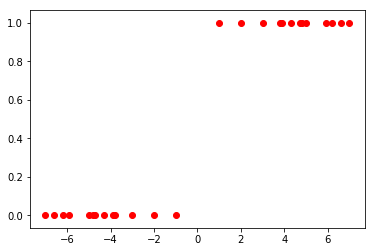Let's look at the sigmoid curve overlaid on these points with increasing values of $\beta$:

In :
beta_0 = 0 # We're only looking at the role of beta_1 here, so we've fixed beta_0
beta_1_choices = [1, 2, 5, 10, 100]

In :
x = np.linspace(-8, 8, 1000)
for beta_1 in beta_1_choices:
y = sigmoid(beta_0 + beta_1 * x)
plt.plot(x, y)
plt.scatter(xs, ys, color = 'r')
plt.show()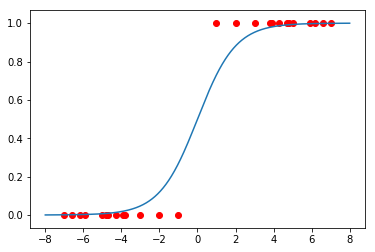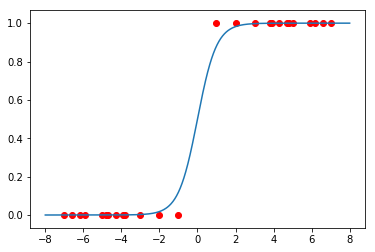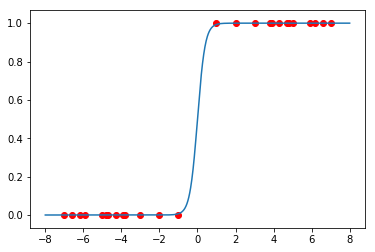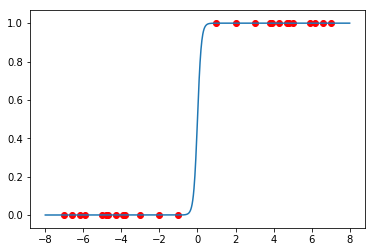/Users/surajrampure/miniconda3/lib/python3.6/site-packages/ipykernel_launcher.py:2: RuntimeWarning: overflow encountered in power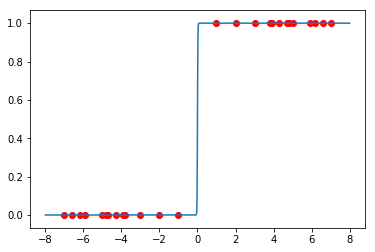Notice, as the size of $\beta_1$ increases, the shape of $\sigma(\beta_0 + \beta_1 x)$ becomes steeper and steeper, and looks closer to a horizontal line. This resembles the hyperplane decision boundary that could be used to separate our data, should it be linearly separable.

This can be rigorously justified by looking at the gradient descent update equation.

### Negative Infinity¶

However, let's now flip our points:

In :
ys_neg = [0 if yi == 1 else 1 for yi in ys]
plt.scatter(xs, ys_neg, color = 'orange');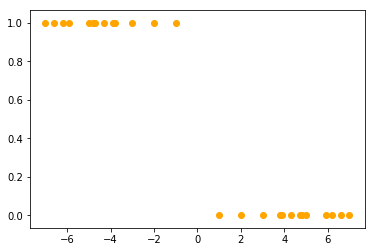And look at decreasing values of beta:

In :
beta_0 = 0
beta_1_choices = [-1, -2, -5, -10, -100]

In :
x = np.linspace(-8, 8, 1000)
for beta_1 in beta_1_choices:
y = sigmoid(beta_0 + beta_1 * x)
plt.plot(x, y)
plt.scatter(xs, ys_neg, color = 'orange')
plt.show()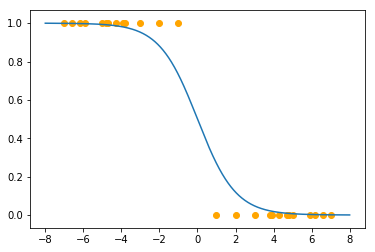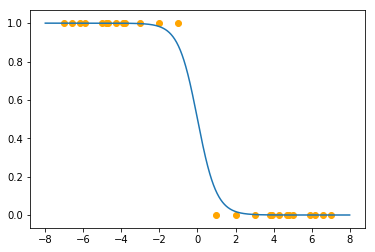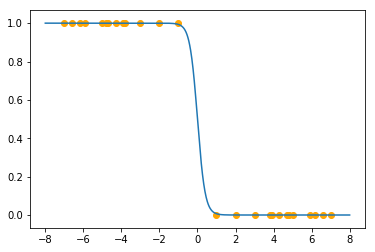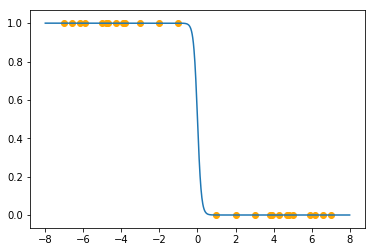/Users/surajrampure/miniconda3/lib/python3.6/site-packages/ipykernel_launcher.py:2: RuntimeWarning: overflow encountered in power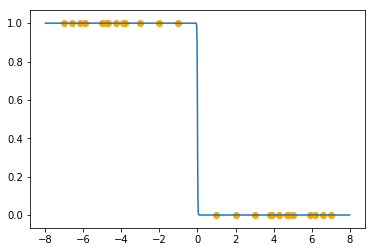Conclusion:

Suppose we have a set of scalars $x$ along with class labels $y$, and our data is linearly separable. Furthermore, suppose our model is $P(Y = 1 \: \big| \: X = x) = \sigma(\beta_0 + \beta_1 x)$. If we can create the following classifier, for some constant $x_0$:

$$classify(x) = \begin{cases} 1 && x \geq x_0 \\ 0 && x < x_0 \end{cases}$$

Then, $\beta_1 \rightarrow \infty$ (or, in terms of past exam questions, "our parameters diverge to positive infinity"). Intuitively speaking, this is the case where all of the class 1 points are "to the right" of all of the class 0 points.

In the opposite case, where we can create the following classifier:

$$classify(x) = \begin{cases} 0 && x \geq x_0 \\ 1 && x < x_0 \end{cases}$$

Then, $\beta_1 \rightarrow - \infty$ (or, again, in terms of past exam questions, "our parameters diverge to negative infinity"). This is the case where all of the class 1 points are "to the left" of all of the class 0 points.

Clarification: Previously, this notebook stated that only $\beta_1$ would approach $\pm \infty$, and not $\beta_0$. This interpretation was not exactly correct, and so I removed any discussion of $\beta_0$ here. @1305 from Spring 2019's Piazza has a nice discussion of this idea (maybe in the future I'll add that information here...)